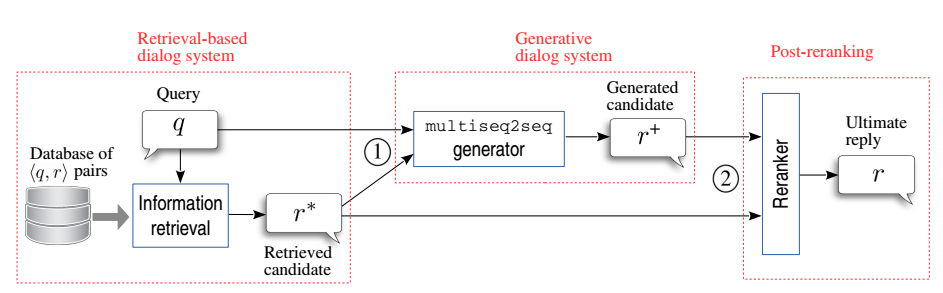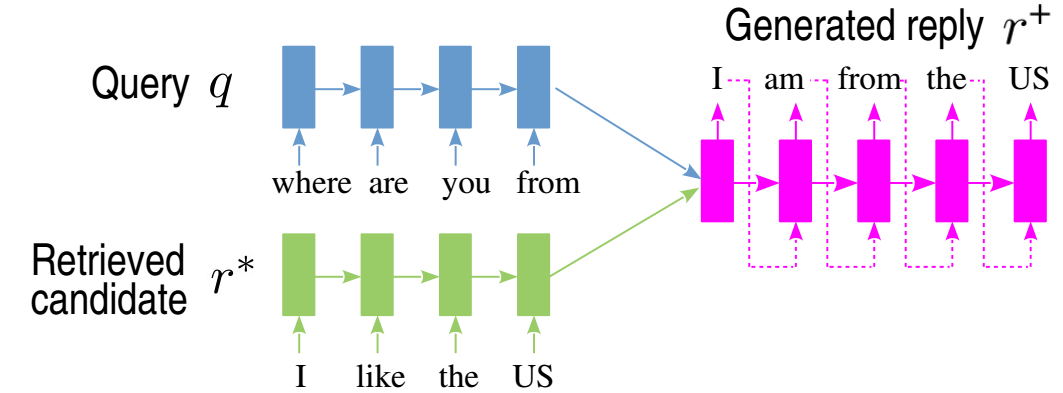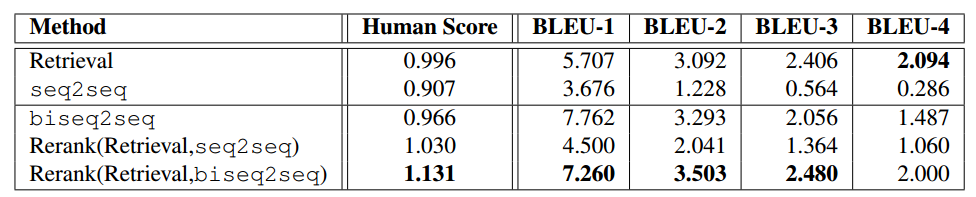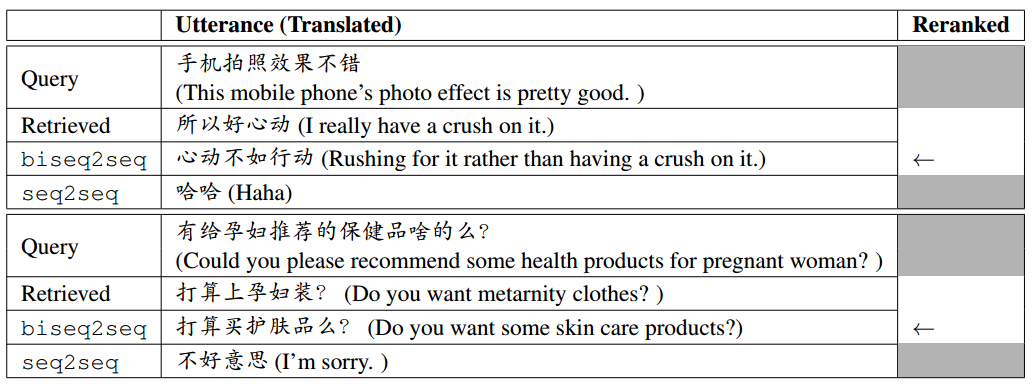# 论文笔记：An Ensemble of Retrieval- and Generation-Based Dialog Systems

1. 使用生成方法时，使用检索到的回复作为额外的信息
2. 对检索方法和生成方法给出的结果，在最后进行重新排序1. 对给定的 query $$q$$，首先从知识库中进行检索，得到候选的回复 $$r^{\ast}$$，这个 $$r^{\ast}$$ 会有很多个
2. 将每个候选回复 $$r^{\ast}$$ 和 $$q$$ 一起输入到生成模型也就是图中的「multiseq2seq generator」中，后文又称之为「biseq2seq」
3. $$q$$ 和 $$r^{\ast}$$ 都是一个句子，它们在 biseq2seq 中经过 encoder 被各自编码成一个向量
4. 将 $$q$$ 和 $$r^{\ast}$$ 的向量 拼接 起来，decoder 以其作为输入，生成一个回复 $$r^{​+}$$
5. 将所有检索出的回复 $$r^{\ast}$$ 和生成的回复 $$r^{​+}$$ 混合起来，进行重排序，返回最优的一个